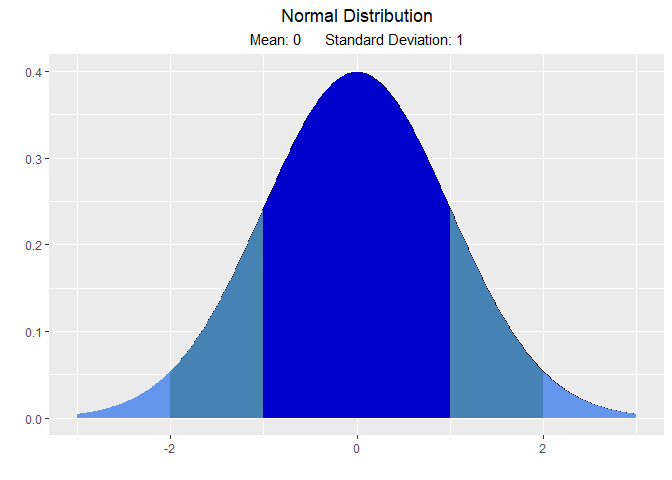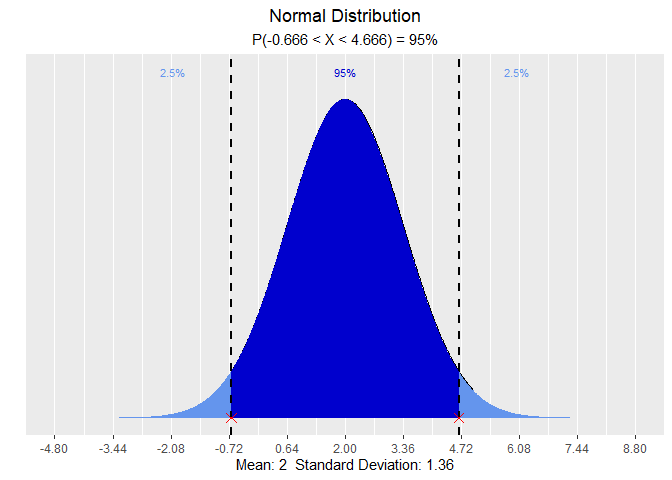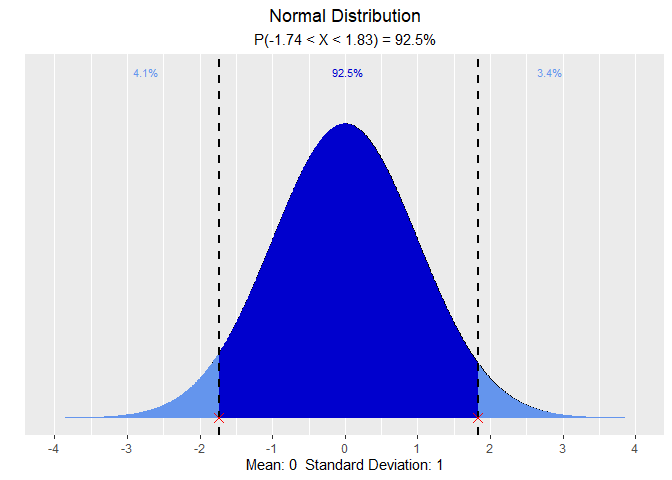# vistributions

Visualize probability distributions

## Installation

``````# Install release version from CRAN
install.packages("vistributions")

# Install development version from GitHub
# install.packages("devtools")

## Usage

### Normal Distribution

``````# visualize normal distribution
vdist_normal_plot()````````````# visualize quantiles out of given probability
vdist_normal_perc(0.95, mean = 2, sd = 1.36, type = 'both')````````````# visualize probability from a given quantile
vdist_normal_prob(c(-1.74, 1.83), type = 'both')``````## Getting Help

If you encounter a bug, please file a minimal reproducible example using reprex on github. For questions and clarifications, use StackOverflow.